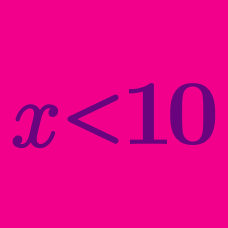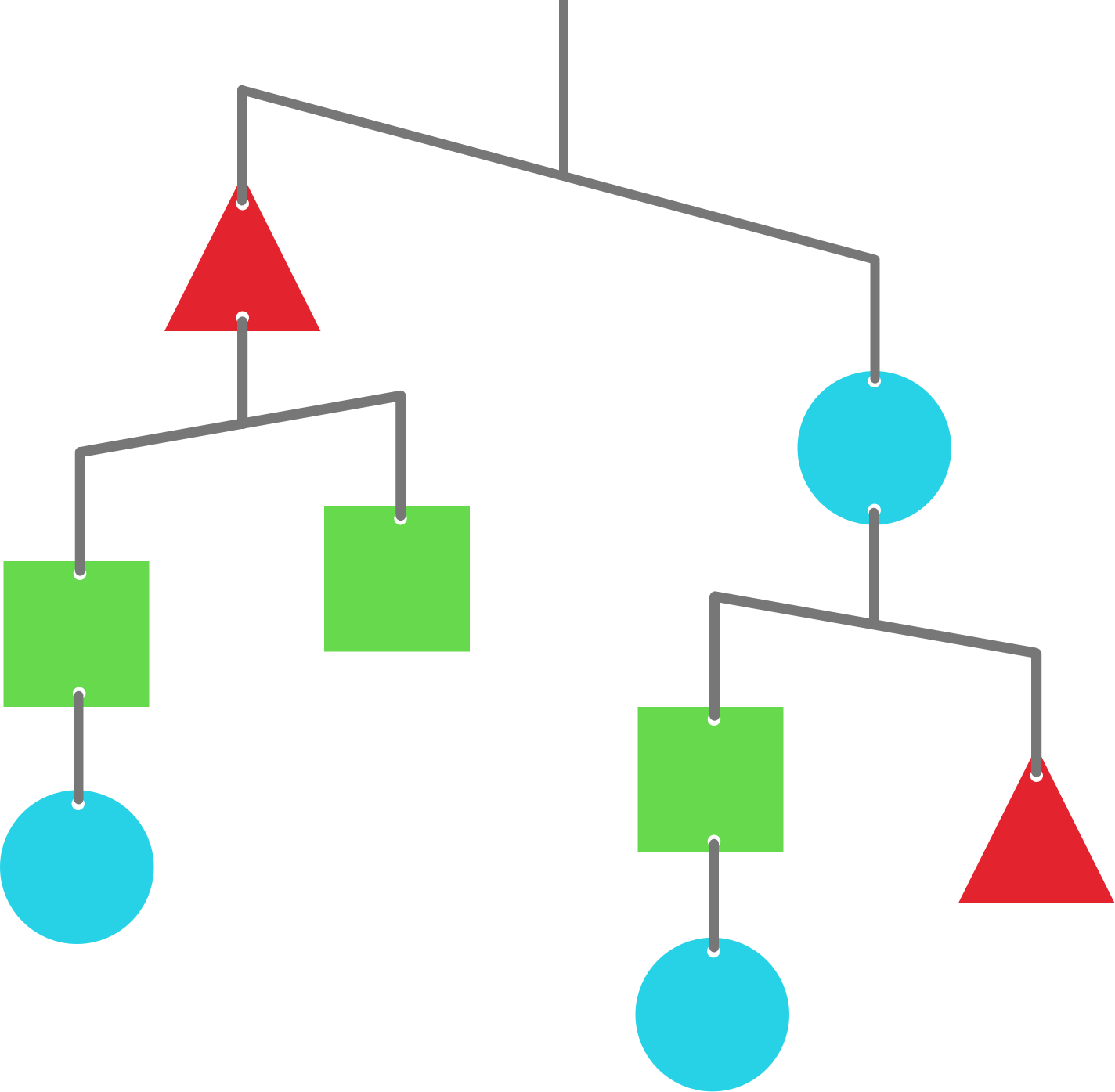Algebra

# Linear Inequalities: Level 2 ChallengesThe above shows how a mobile will be balanced when left to hang. Assume that the fulcrum is at the center of each rod.

What are the relative weights of these shapes?

If $-2 < x < 2$ and $-5 < y < 5$, what is the range of all possible values of $y - x$?

If $\color{#D61F06}{a}$ and $\color{#3D99F6}{b}$ are non-zero real numbers with $\color{#D61F06}{a}>\color{#3D99F6}{b},$ then $\dfrac{1}{\color{#D61F06}{a}} < \dfrac{1}{\color{#3D99F6}{b}}$.

$\large \color{#3D99F6}{\large{A=\frac{15^{16} +1}{15^{17} +1}} \quad {\color{#333333}{\text{or}}} \quad \color{#D61F06}{B=\frac{15^{15} +1}{15^{16} +1}}}$

Which is larger?

Amy and Brian go to a shop to buy a banana. However, Amy is 2 pennies short, and Brian is 24 pennies short. Even if they combine their money, they still can't afford the banana!

How many pennies does the banana cost?

(You should assume that Amy's money, Brian's money, and the cost of the banana are all positive integer amounts of pennies.)

×

Problem Loading...

Note Loading...

Set Loading...